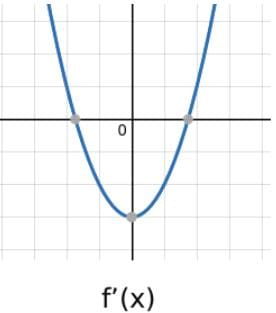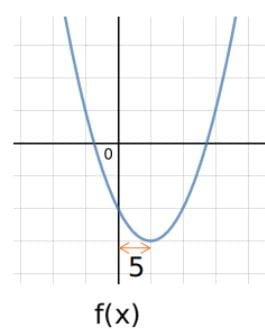A quadratic equation f(x) = 0 has one positiv...
A quadratic equation f(x) = 0 has one positive and one negative root. If the graph of f(x) is shifted left along the x-axis to form f'(x) in such a way that the sum of roots of the quadratic equation f'(x) = 0 is zero, the distance between the minima of f(x) and f'(x) is 5 units. Which of the following is a possible sum of the roots of the equation f(x) = 0?
• a)
10
• b)
5
• c)
2.5
• d)
-2.5
A quadratic equation f(x) = 0 has one positive and one negative root. ...
Let the roots of f'(x) = -a,a
The distance between the minima of f(x) and f'(x) = 5. This implies that f(x) has been shifted 5 units towards the left to get f'(x).
Hence, the roots of f(x) = -a+5 and a+5.
Hence, sum of roots of f(x) = -a+5+a+5 = 10 units.1 Crore+ students have signed up on EduRev. Have you?
1 Crore+ students have signed up on EduRev. Have you?

### Learn this topic in detailCAT Mock Test- 6 66 Ques | 120 MinsMore from Related Course Quantitative Aptitude (Quant)View courses related to this question

### Quick links for CAT exam850+
Video Lectures2500+
Revision Notes600+
Online Tests34+
Courses
A quadratic equation f(x) = 0 has one positive and one negative root. If the graph of f(x) is shifted left along the x-axis to form f(x) in such a way that the sum of roots of the quadratic equation f(x) = 0 is zero, the distance between the minima of f(x) and f(x) is 5 units. Which of the following is a possible sum of the roots of the equation f(x) = 0?a)10b)5c)2.5d)-2.5Correct answer is option 'A'. Can you explain this answer?
Question Description(Scan QR code)